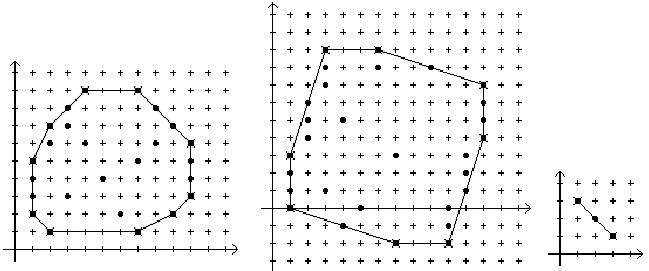Online JudgeProblem SetAuthorsOnline ContestsUser
Web Board
F.A.Qs
Statistical Charts
Problems
Submit Problem
Online Status
Prob.ID:
Register
Authors ranklist
Current Contest
Past Contests
Scheduled Contests
Award Contest
Register
Language:
Convex Hull of Lattice Points
 Time Limit: 1000MS Memory Limit: 65536K Total Submissions: 650 Accepted: 355

Description

A lattice point is a point with integer coordinates. A lattice polygon is a polygon with all vertices lattice points.

A polygon is convex if any line segment between two points of the polygon is inside (or on the boundary of) the polygon. Equivalently, the interior angle at each polygon vertex is less than 180 degrees.

For a set S, of lattice points, the convex hull is the smallest convex (lattice) polygon which contains all points of the set. (The vertices of the convex hull must be members of the set of lattice points). If all the points are on a single straight line, the convex hull will be a line segment (a degenerate polygon – see rightmost diagram below). In the diagrams below, the points of the set are indicated by solid dots, the vertices of the convex hull by X’s and the convex hull is drawn connecting the vertices. Note that not all points on the convex hull polygon are vertices.The vertices of a lattice polygon are in standard order if:
a) The first vertex is the one with the largest y value. If two vertices have the same y value, the one with the smaller x value is the first.
b) Vertices are given in clockwise order around the polygon.

Write a program that reads a set of lattice points and outputs the vertices of the convex hull of the points in standard order.

Input

The first line of input contains a single integer P, (1 ≤ P ≤ 1000), which is the number of data sets that follow. The first line of each data set contains the data set number, followed by a space, followed by a decimal integer giving the number of points N, (3 ≤ N ≤ 50), in the set. The remaining lines in the data set contain the points in the set, at most 5 points per line (the last line may have fewer). Each point consists of 2 space separated decimal integer values representing the x and y coordinates respectively.

Output

For each data set there are multiple lines of output. The first line contains a decimal integer giving the data set number followed by a single space, followed by a decimal integer giving the total number of vertices of the convex hull. The vertices of the convex hull follow, one per line in standard order. Each line contains the decimal integer x coordinate, a single space and the decimal integer y coordinate.

Sample Input

```4
1 25
2 1 7 1 1 2 9 2 1 3
10 3 1 4 10 4 1 5 10 5
2 6 10 6 2 7 9 7 3 8
8 8 4 9 7 9 6 2 3 3
5 4 7 5 8 6 4 6 3 7
2 30
3 9 6 9 3 8 9 8 3 7
12 7 2 6 12 6 2 5 12 5
2 4 12 4 1 3 11 3 1 2
11 2 1 1 11 1 1 0 10 0
4 -1 10 -1 7 -2 10 -2 5 0
7 3 4 5 6 8 3 1 2 6
3 3
3 1 2 2 1 3
4 6
1 3 19 1 4 2 2 1 11 2
10 1```

Sample Output

```1 10
4 9
7 9
10 6
10 3
9 2
7 1
2 1
1 2
1 5
2 7
2 8
3 9
6 9
12 7
12 4
10 -2
7 -2
1 0
1 3
3 2
1 3
3 1
4 4
1 3
11 2
19 1
2 1```

Source

[Submit]   [Go Back]   [Status]   [Discuss]Home PageGo BackTo top